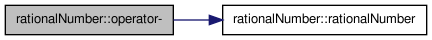# rationalNumber Class Reference [Algebra objects, Field and Ring elements]

represents a rational number. Value range of the numerator and nominator is shrinked to a range of an 'int' More...

`#include <rationalNumber.h>`

Collaboration diagram for rationalNumber:[legend]

List of all members.

## Public Member Functions

Constructors

rationalNumber ()
initialize rational number with zero
rationalNumber (int value)
initialize rational number with value
rationalNumber (int _numerator, int _denominator)
rationalNumber (std::stringstream &number)
Data Access

int getNumerator () const
int getDenominator () const
Properties

bool isZero () const
bool isNotZero () const
int getSign () const
Operators

rationalNumberoperator+= (const rationalNumber &r2)
std::ostream & operator<< (std::ostream &os) const
bool operator== (const rationalNumber &r2) const
bool operator!= (const rationalNumber &r2) const
rationalNumber operator- ()

## Static Public Member Functions

static bool memsetClearAllowed ()

## Static Public Attributes

static const rationalNumber Zero
static const rationalNumber One

## Protected Member Functions

int extractSign (std::stringstream &number)
liest auch +- als '-' Merkt aber nicht, dass '+-+' '-+-' o.Ä Unsinn ist.

## Protected Attributes

Data

int numerator
int denominator
denomitator; is always kept positive.

## Detailed Description

Todo:
replace internal int topy by int32 oder int64

Definition at line 22 of file rationalNumber.h.

## Constructor & Destructor Documentation

 rationalNumber::rationalNumber ( ) ` [inline]`

Definition at line 97 of file rationalNumber.h.

References denominator, and numerator.

Referenced by operator-().

 rationalNumber::rationalNumber ( int value ) ` [inline]`

Definition at line 104 of file rationalNumber.h.

References denominator, and numerator.

 rationalNumber::rationalNumber ( int _numerator, int _denominator ) ` [inline]`

Definition at line 110 of file rationalNumber.h.

References denominator, and numerator.

 rationalNumber::rationalNumber ( std::stringstream & ssNum )

extract rational number from stream. Format: {+|-}sign[variable] | {+|-}[sign}(nominator){'/'{sign}denominator}}[variable]. Braces are not allowed.

Todo:
ueberlegen, wie verhindert werden soll, dass '-' als '-1' gelesen wird, wenn nicht '-x' oder ä. folgt.

Definition at line 15 of file rationalNumber.cc.

References denominator, extractChar(), extractSign(), getSign(), and numerator.

Here is the call graph for this function:## Member Function Documentation

 int rationalNumber::extractSign ( std::stringstream & number ) ` [protected]`

Definition at line 86 of file rationalNumber.cc.

Referenced by rationalNumber().

 int rationalNumber::getDenominator ( ) const` [inline]`

Definition at line 140 of file rationalNumber.h.

References denominator.

 int rationalNumber::getNumerator ( ) const` [inline]`

Definition at line 135 of file rationalNumber.h.

References numerator.

 int rationalNumber::getSign ( ) const` [inline]`

Definition at line 124 of file rationalNumber.h.

References denominator, and numerator.

Referenced by rationalNumber().

 bool rationalNumber::isNotZero ( ) const` [inline]`

Definition at line 150 of file rationalNumber.h.

References numerator.

 bool rationalNumber::isZero ( ) const` [inline]`

Definition at line 145 of file rationalNumber.h.

References numerator.

 static bool rationalNumber::memsetClearAllowed ( ) ` [inline, static]`

Definition at line 39 of file rationalNumber.h.

 bool rationalNumber::operator!= ( const rationalNumber & r2 ) const

Definition at line 138 of file rationalNumber.cc.

References denominator, and numerator.

 rationalNumber & rationalNumber::operator+= ( const rationalNumber & r2 )

Definition at line 120 of file rationalNumber.cc.

References denominator, and numerator.

 rationalNumber rationalNumber::operator- ( ) ` [inline]`

Definition at line 79 of file rationalNumber.h.

References denominator, numerator, and rationalNumber().

Here is the call graph for this function:std::ostream & rationalNumber::operator<< ( std::ostream & os ) const

Definition at line 144 of file rationalNumber.cc.

References denominator, and numerator.

 bool rationalNumber::operator== ( const rationalNumber & r2 ) const

Definition at line 133 of file rationalNumber.cc.

References denominator, and numerator.

## Member Data Documentation

 int rationalNumber::denominator` [protected]`
 int rationalNumber::numerator` [protected]`

Definition at line 29 of file rationalNumber.h.

 const rationalNumber rationalNumber::One` [static]`

Definition at line 37 of file rationalNumber.h.

 const rationalNumber rationalNumber::Zero` [static]`

Definition at line 36 of file rationalNumber.h.

The documentation for this class was generated from the following files:
Generated on Tue Nov 23 13:10:53 2010 for centerfocus by1.6.3